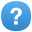Add option to NumberFormatter to select different notations in different ranges

Description

For example, in printf, you can use `%g` to use simple notation for small numbers and scientific notation for large numbers. There should be a way to do this in NumberFormatter. Ideas:

```1 2 ``````Notation.scientific().exceptWithinRange(1e-6, 1e6) Notation.range(Notation.scientific(), 1e-6, Notation.simple(), 1e6, Notation.scientific())```

We also need to define exactly what happens at the boundary. For example, if you say to use scientific notation above 1e6, and you ask us to format 999999 with 3 significant digits, do we use simple notation because the number is less than 1e6, or do we use scientific notation since the rounded value is at the threshold?

Shane Carr

Shane Carr

Days

Priorityassess